# Algebra II : Range

## Example Questions

1 2 3 4 5 6 7 8 10 Next →

### Example Question #91 : Range

Determine the range of the data set: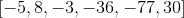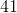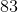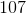Explanation:

The range of the numbers is the difference between the highest and lowest numbers provided.

The largest number is.

The smallest number is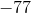.

Subtract both values.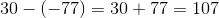The answer is:### Example Question #92 : Range

Determine the range of the numbers: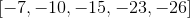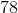Explanation:

The range of the numbers is the difference between the largest and smallest numbers.

The largest number is:The smallest number is: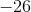Subtract the quantity of both numbers.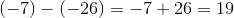The answer is:### Example Question #93 : Range

Determine the range of the following numbers: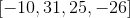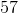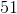Explanation:

The range is the difference between the highest and lowest numbers.

Identify the highest and lowest numbers.

The highest number is:The lowest number is:Subtract both numbers.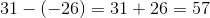The answer is:### Example Question #94 : Range

The 2014 Sinquefield Cup consisted of nine total rounds. In this chess tournament, a win is worth one point, a draw is worth half a point, and a loss is worth no point. At the end of the tournament, the player with the highest score wins the tournament. Below are the scores of the players.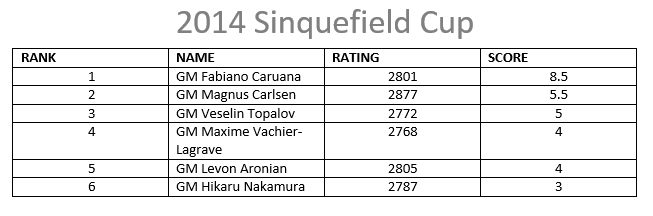Find the range of the scores of the players.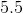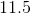The range cannot be calculated.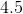Explanation:

The range can be found by subtracting the smallest value from the largest value.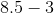Solution: 5.5

### Example Question #95 : Range

What is the range of the following data set: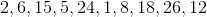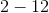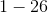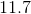Cannot be determined from above informationThe range corresponds the lowest and highest values in a data set. In this case, that corresponds toand.corresponds to the median.corresponds to the mean.corresponds to the first and last numbers in the set. Each of these options are incorrect.